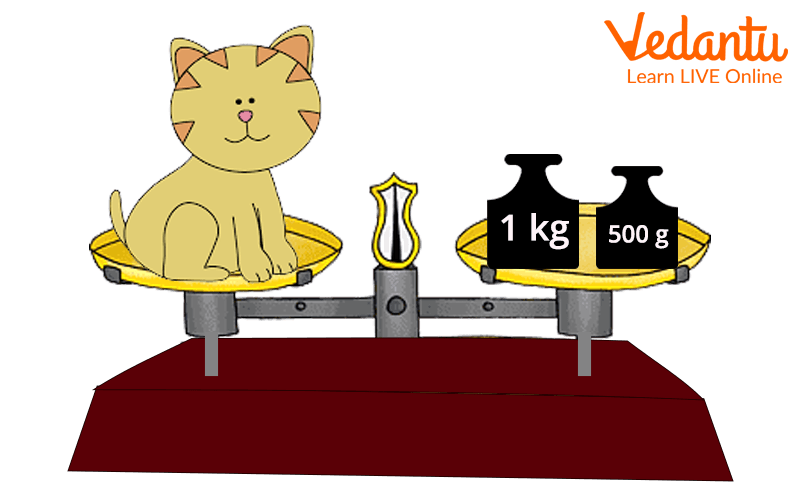Courses
Courses for Kids
Free study material
Free LIVE classes
More

# Expressing Weight as kg Using DecimalsLIVE
Join Vedantu’s FREE Mastercalss

## Conversion Between Units

Conversion between units of mass involves moving the decimal point to the right or the left. To measure something, you need a system of units. The decimal metric is a system of units in which multiples or partial multiples of a unit of measure are connected by multiples.

## What are Mass and Weight?

Mass and weight are closely related. Weight is a measure of how strongly the Earth pulls the object. Therefore, objects in space weigh less than the Earth's surface.

However, the amount of material object remains the same; this is known as the mass of the object. The basic unit of mass in the metric system is the kilogram.Expressing Weight Using a Example

## What Is Decimal?

Decimals are numbers that have an integral part and a fractional part separated by a decimal point. The integral part is those parts whose value can be negative or positive such as 5 and -4. The fractional part is those whose value lies between zero and one such as 0.25. In decimal numbers the fractional part is on the right side of the decimal point and on the left side of the decimal it is the integral part.

## Units of Mass

A system of measurement in which the basic units are meters, seconds, and kilograms is called metric units.

Conversion of the unit of mass is given as follows:

 1 milligram 0.001 gram 1 centigram 0.01 gram 1 decigram 0.1 gram 1 decagram 10 gram 1 hectogram 100 gram 1 kilogram 1000 grams

## Conversion of Unit of Mass

The mass when changed from a smaller to a larger unit needs to be written in a decimal form. This is done by simple division. Let's understand this by an example where you need to change 683g into kg. To express as kg using decimals we need to know the basic conversion which is 1000 g is equal to 1 kg. so, we will divide 683 by 1000 and get 0.683. So, 683 gm is equal to 0.683kg.

To change the mass from a larger unit to smaller unit, we need to multiply by the 10,100 or 1000 depending on the unit of conversion.

For example, if we need to express 75 hectogram into gram then we will multiply 75 by 100 as 1 hectogram is equal to 100gm. This will result in 7500gm.

## Conclusion

Unit conversion is a multi-step procedure that requires multiplying or dividing by a numerical number or, more specifically, a conversion factor. The technique could also require selecting the appropriate number of critical digits and rounding. Different conversion units are applied to evaluate different parameters.

## Solved Examples

1. Which one is greater, 45kg or 350gm?

a. 45kg

b. 350gm

c. none of the above

d. cannot decide

Ans: 45kg

Explanation: If we change the units of conversion into the same units of both the numbers then it would be easy to determine which one is greater. So 45kg can be written as 45000gm. If we compare 45000gm and 350gm then 45000gm is greater.

2. Which is the smaller unit hectogram or centigram?

a. Hectogram

b. centigram

c. both

d. None of the above

Ans: Centigram

Explanation: Centigram is the smallest unit as 1 centigram is equal to 0.01gm and a hectogram is equal to 100gm.

3. Which is the smallest unit of mass?

a. kilogram

b. centigram

c. gram

d. hectogram

Ans: Gram

The Smallest unit of mass in gram. One kilogram is equal to 1000 grams. Hence, grams are the smallest unit of mass in grams.

Last updated date: 02nd Oct 2023
Total views: 68.1k
Views today: 2.68k

## FAQs on Expressing Weight as kg Using Decimals

1. What is the Aim of Unit Conversion?

Unit conversion is critical since not all nations use the standard metric system and have distinct units for measuring length, weight, area, and so on. As a result, converting units becomes the primary concern, and unit conversion serves the goal.

2. How Do You Convert One Unit to Another?

For unit conversions, the following procedures are taken:

Step 1: Convert the value to a fraction.

Step 2: Multiply or divide as necessary.

Cancel the units in step three (same units from top and bottom)

Step 4: Write the simplified solution in the appropriate unit.

3. 456gm - Express in decimal form.

As 456g can be converted into kilogram and be written as a decimal like 0.456kg.

4. Express 8 kg 8 g as kg using decimals.

As 1000 g is equal to 1 kg then 8g will be 0.008kg. This can be added to 8kg. We will get the answer as 8.008kg.